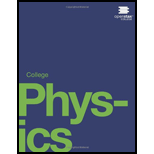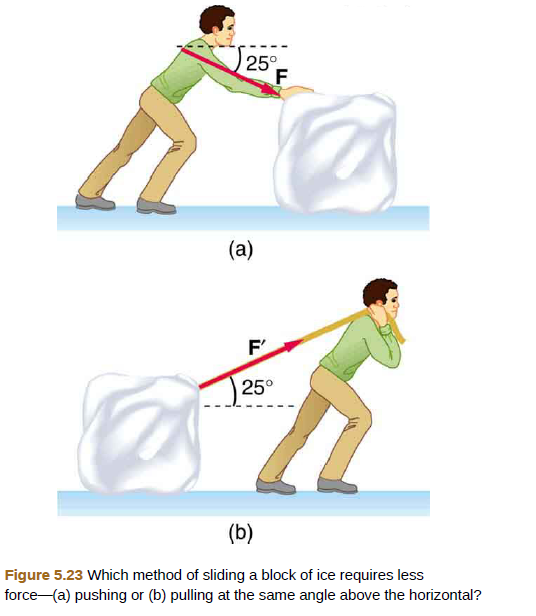# Repeat Exercise 5.18 with the contestant pulling the block of ice with a rope over his shoulder at the same angle above the horizontal as shown in Figure 5.23(b).### College Physics

1st Edition
Paul Peter Urone + 1 other
Publisher: OpenStax College
ISBN: 9781938168000### College Physics

1st Edition
Paul Peter Urone + 1 other
Publisher: OpenStax College
ISBN: 9781938168000

#### Solutions

Chapter
Section
Chapter 5, Problem 19PE
Textbook Problem

## Repeat Exercise 5.18 with the contestant pulling the block of ice with a rope over his shoulder at the same angle above the horizontal as shown in Figure 5.23(b).Expert Solution
To determine

(a)

The minimum force must exert to get the block moving

### Explanation of Solution

Given info:

Mass of the block, m=45kg

Diagram of the block

Figure.1

Formula used:

For a stationary object algebraic sum of all the forces in a direction must be zero.

Fx=0,&Fy=0

Calculation:

The weight of the block will act in downward direction

W=mg=45×9.81=441.45N

Consider the free body diagram of the block

Figure.2

The normal force on the lake by the block is

N=mgFsin25οN=441

Expert Solution
To determine

(b)

Acceleration of the block once it start moving

### Want to see the full answer?

Check out a sample textbook solution.See solution

### Want to see this answer and more?

Bartleby provides explanations to thousands of textbook problems written by our experts, many with advanced degrees!

See solution